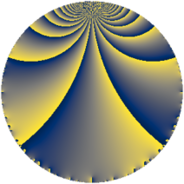Properties

 Label 1859.4.hLevel $1859$ Weight $4$ Character orbit 1859.h Rep. character $\chi_{1859}(170,\cdot)$ Character field $\Q(\zeta_{5})$ Dimension $1816$ Sturm bound $728$

Related objects

Defining parameters

 Level: $$N$$ $$=$$ $$1859 = 11 \cdot 13^{2}$$ Weight: $$k$$ $$=$$ $$4$$ Character orbit: $$[\chi]$$ $$=$$ 1859.h (of order $$5$$ and degree $$4$$) Character conductor: $$\operatorname{cond}(\chi)$$ $$=$$ $$11$$ Character field: $$\Q(\zeta_{5})$$ Sturm bound: $$728$$

Dimensions

The following table gives the dimensions of various subspaces of $$M_{4}(1859, [\chi])$$.

Total New Old
Modular forms 2240 1904 336
Cusp forms 2128 1816 312
Eisenstein series 112 88 24

Trace form

 $$1816 q - q^{2} + 3 q^{3} - 1759 q^{4} - q^{5} - 55 q^{6} - 29 q^{7} + 21 q^{8} - 3947 q^{9} + O(q^{10})$$ $$1816 q - q^{2} + 3 q^{3} - 1759 q^{4} - q^{5} - 55 q^{6} - 29 q^{7} + 21 q^{8} - 3947 q^{9} + 8 q^{10} + 31 q^{11} + 114 q^{12} - 50 q^{14} - 55 q^{15} - 6883 q^{16} + 67 q^{17} - 12 q^{18} - 30 q^{19} - 470 q^{20} + 98 q^{21} - 511 q^{22} - 404 q^{23} - 561 q^{24} - 10207 q^{25} + 246 q^{27} - 252 q^{28} - 201 q^{29} + 402 q^{30} - 143 q^{31} + 332 q^{32} - 1746 q^{33} + 1618 q^{34} - 589 q^{35} - 12124 q^{36} + 423 q^{37} - 560 q^{38} - 130 q^{40} - 1485 q^{41} + 48 q^{42} + 1350 q^{43} - 1202 q^{44} - 676 q^{45} + 2346 q^{46} - 465 q^{47} + 1330 q^{48} - 18287 q^{49} - 843 q^{50} - 860 q^{51} - 123 q^{53} + 3384 q^{54} - q^{55} + 44 q^{56} - 768 q^{57} + 1958 q^{58} - 418 q^{59} + 1516 q^{60} + 525 q^{61} + 3102 q^{62} - 5264 q^{63} - 23587 q^{64} - 6200 q^{66} - 3538 q^{67} - 1582 q^{68} + 1182 q^{69} + 292 q^{70} - 3519 q^{71} - 1811 q^{72} - 3397 q^{73} + 2588 q^{74} + 582 q^{75} + 2866 q^{76} - 93 q^{77} + 3657 q^{79} + 10054 q^{80} - 22940 q^{81} - 1495 q^{82} - 1418 q^{83} + 5296 q^{84} + 1601 q^{85} + 375 q^{86} - 4790 q^{87} - 5623 q^{88} - 3970 q^{89} - 3582 q^{90} + 370 q^{92} + 3121 q^{93} - 2596 q^{94} + 1425 q^{95} - 10988 q^{96} - 642 q^{97} + 4320 q^{98} + 1507 q^{99} + O(q^{100})$$

Decomposition of $$S_{4}^{\mathrm{new}}(1859, [\chi])$$ into newform subspaces

The newforms in this space have not yet been added to the LMFDB.

Decomposition of $$S_{4}^{\mathrm{old}}(1859, [\chi])$$ into lower level spaces

$$S_{4}^{\mathrm{old}}(1859, [\chi]) \cong$$ $$S_{4}^{\mathrm{new}}(11, [\chi])$$$$^{\oplus 3}$$$$\oplus$$$$S_{4}^{\mathrm{new}}(143, [\chi])$$$$^{\oplus 2}$$